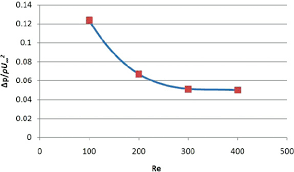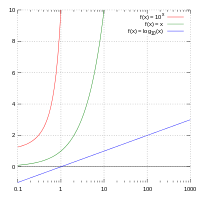## How to Solve and Calculate for a Dimensionless Pressure Drop, Oil Rate, Oil FVF, Oil Viscosity in Well Testing | The Calculator EncyclopediaThe image above represents dimensionless pressure drop.

To compute for the dimensionless pressure drop, five essential parameters are needed and these parameters are Permeability-Thickness Product (kh), Pressure Drop (ΔP), Oil Rate (Qo), Oil Viscosity (μo) and Oil FVF (Bo).

The formula for calculating dimensionless pressure drop:

PD = khΔP / 141.2QoBoμo

Where;

PD = Dimensionless Pressure Drop
kH = Permeability-Thickness Product
ΔP = Pressure Drop
Qo = Oil Rate
μo = Oil Viscosity
Bo = Oil FVF

Let’s solve an example;
Find the dimensionless pressure drop with a permeability-thickness product of 70, a pressure drop of 30, an oil rate of 49, an oil viscosity of 19 and oil FVF of 31.

This implies that;

kh = Permeability-Thickness Product = 20
ΔP = Pressure Drop = 30
Qo = Oil Rate = 49
μo = Oil Viscosity = 19
Bo = Oil FVF = 31

PD = khΔP / 141.2QoBoμo
PD = 20 x 30/141.2 x 49 x 31 x 19
PD = 20 x 30/4075173.19
PD = 600/4075173.19
PD = 0.000147

Therefore, the dimensionless pressure drop is 0.000147 psi.

Calculating the Permeability-Thickness Product when Dimensionless Pressure Drop, Pressure Drop, Oil Rate, Oil Viscosity and Oil FVF.

kh = PD x 141.2QoBoμo / ΔP

Where;

kh = Permeability-Thickness Product
PD = Dimensionless Pressure Drop
ΔP = Pressure Drop
Qo = Oil Rate
μo = Oil Viscosity
Bo = Oil FVF

Let’s solve an example;
Find the permeability-thickness product with a dimensionless pressure drop of 28, a pressure drop of 16, an oil rate of 21, an oil viscosity of 18 and oil FVF of 24 .

This implies that;

PD = Dimensionless Pressure Drop = 28
ΔP = Pressure Drop =16
Qo = Oil Rate = 21
μo = Oil Viscosity = 18
Bo = Oil FVF = 24

kh = PD x 141.2QoBoμo / ΔP
kh = 28 x 141.2 x 21 x 24 x 18 / 16
kh = 35867059.8 / 16
kh = 2241691.2

Therefore, the permeability-thickness product is 2241691.2.

Calculating the Pressure Drop when Dimensionless Pressure Drop, Permeability-Thickness Product, Oil Rate, Oil Viscosity and Oil FVF.

ΔP = PD x 141.2QoBoμo / kh

Where;

ΔP = Pressure Drop
PD = Dimensionless Pressure Drop
kh = Permeability-Thickness Product
Qo = Oil Rate
μo = Oil Viscosity
Bo = Oil FVF

Let’s solve an example;
Find the pressure drop with a dimensionless pressure drop of 38, a permeability-thickness product of 24, an oil rate of 11, an oil viscosity of 8 and oil FVF of 12.

This implies that;

PD = Dimensionless Pressure Drop = 38
kh = Permeability-Thickness Product = 24
Qo = Oil Rate = 11
μo = Oil Viscosity = 8
Bo = Oil FVF = 12

ΔP = PD x 141.2QoBoμo / kh
ΔP = 38 x 141.2 x 11 x 12 x 8 / 24
ΔP = 5666073.6 / 24
ΔP = 236086.4

Therefore, the pressure drop is 236086.4.

## How to Calculate and Solve for Semi-Log Pressure-Time Slope, Permeability-Thickness Product, Oil Rate, Oil FVF, Oil Viscosity in Well Testing | Nickzom CalculatorThe image above represents semi-log pressure-time slope.

To compute for the semi-log pressure-time slope, four essential parameters are needed and these parameters are Permeability-Thickness Product (kh), Oil Rate (Qo), Oil Viscosity (μo) and Oil FVF (Bo).

The formula for calculating the semi-log pressure-time slope:

m = 162.6QoBoμo / kh

Where;

m = Semi-Log Pressure-Time Slope
kh = Permeability-Thickness Product
Qo = Oil Rate
μo = Oil Viscosity
Bo = Oil FVF

Let’s solve an example;
Find the semi-log pressure-time slope, when permeability-thickness product is 12, oil rate is 17, oil viscosity is 21 and oil FVF is 13.7.

This implies that;

kh = Permeability-Thickness Product = 12
Qo = Oil Rate = 17
μo = Oil Viscosity = 21
Bo = Oil FVF = 13.7

m = 162.6QoBoμo / kh
m = 162.6 x 17 x 13.7 x 21 / 12
m = 795260.34 / 12
m = 66271.69

Therefore, the semi-log pressure-time slope is 66271.69.

Calculating the Permeability-Thickness Product when Semi-Log Pressure-Time Slope, Oil Rate, Oil Viscosity and Oil FVF is Given.

kh = 162.6QoBoμo / m

Where;

kh = Permeability-Thickness Product
m = Semi-Log Pressure-Time Slope
Qo = Oil Rate
μo = Oil Viscosity
Bo = Oil FVF

Let’s solve an example;
Find the permeability-thickness product, when semi-log pressure-time slope is 90, oil rate is 23, oil viscosity is 27 and oil FVF is 14.

This implies that;

m = Semi-Log Pressure-Time Slope = 90
Qo = Oil Rate = 23
μo = Oil Viscosity = 27
Bo = Oil FVF = 14

kh = 162.6QoBoμo / m
kh = 162.6 x 23 x 14 x 27 / 90
kh = 1413644.4 / 90
kh = 15707.16

Therefore, the permeability-thickness product is 15707.16.

Calculating the Oil Rate when Semi-Log Pressure-Time Slope, Permeability-Thickness Product, Oil Viscosity and Oil FVF is Given.

Qo = m x kh / 162.6Boo

Where;

Qo = Oil Rate
m = Semi-Log Pressure-Time Slope
kh = Permeability-Thickness Product
μo = Oil Viscosity
Bo = Oil FVF

Let’s solve an example;
Find the oil rate when semi-log pressure-time slope is 110, permeability-thickness product is 42, oil viscosity is 31 and oil FVF is 11.

This implies that;

m = Semi-Log Pressure-Time Slope = 110
kh = Permeability-Thickness Product = 42
μo = Oil Viscosity = 31
Bo = Oil FVF = 11

Qo = m x kh / 162.6Boμo
Qo = 110 x 42 / 162.6 x 11 x 31
Qo = 4620 / 55446.6
Qo = 0.0833

Therefore, the oil rate is 0.0833.